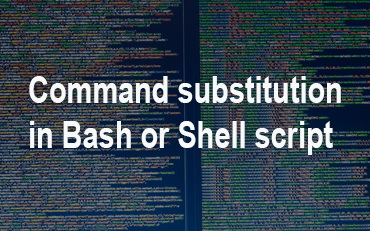# What does \${} and \$() mean in Shell Script

Every Linux has a powerful shell that is used to run commands and scripts. But most often, beginners find it difficult to substitute the value of a shell variable in another shell script of command, using parameter substitution \${}. They also find it difficult to substitute another command in a shell script, using command substitution \$(). In this article, we will learn what does

## What does \${} and \$() mean in Shell Script

Command substitution and Parameter substitution are two most powerful features in shell script.

## Command Substitution/Expansion

Command substitution allows you to replace a command in shell script, with the output of the command. Here is the syntax for it.

```\$(command)
or
`command````

Here is a simple example to substitute date command’s output as their input.

```\$ echo \$(date)
\$ echo `date````

You can also assign the output of command substitution to another variable. Here is an example where we assign value of date command to to TODAY variable.

```\$ TODAY=\$(date)
\$ echo "\$TODAY"
```

You can also use command substitution in shell loops. Here is an example to loop through all the .txt files in a specific folder and print the contents of each file.

```for f in /home/*.txt
do
echo "\$f"
done```

You can also use command substitution in shell script. Here is an example where we have simply included all the above shell commands in a shell script. First we create an empty shell script file commands.sh

`\$ vi commands.sh`

Next add the following lines to it.

```#!/bin/sh

\$ echo \$(date)
\$ echo `date`

\$ TODAY=\$(date)
\$ echo "\$TODAY"

for f in /home/*.txt
do
echo "\$f"
done```

Save and close the file. Make it an executable.

`\$ chmod +x commands.sh`

Run the shell script with the following command.

`\$ ./commands.sh`

## Parameter Substitution/Expansion

Parameter substitution is when we simply substitute the variable with its value. It is similar to command substitution but in this case, it is a direct substitution without any evaluation, as done in the case of command substitution. Here is the syntax for parameter substitution/expansion.

`\${parameter}`

Here is an example of parameter expansion where we substitute the value of variable name with its value.

```\$ name="john doe"
\$ echo "\${name}"```

You may be wondering what is the difference between \$variable and \${variable} since \$variable also replaces variable with its value. Using curly braces helps shell correctly interpret the variable in case there is something that immediately follows the variable. Let us explain with an example. Look at the following command.

```\$ name="john doe"
\$ echo "The name of the person is \$name_"
```

The output of above echo command will be

`The name of the person is `

It will not substitute the value of variable \$name in the echo command. This is because shell looks for variable name_ and not name. If you run the following command

`\$ echo "The name of the person is \${name}_"`

it will correctly do the variable expansion.

`The name of the person is john doe.`

You can also set default values for variable substitution such that if it has null value, then the default value is substituted.

In this article, we have learnt about command substitution as well as parameter substitution. They are both amazing features of Linux shell and help you save a lot of code. But be careful with its syntax, since changing one character with another will completely change the meaning of the expression. For example, using round braces instead of curly braces, or using – instead of + can lead to completely different results.